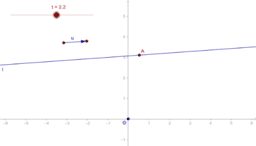# Vectors 2D (Two-Dimensional)

Parent topic:
Vectors
•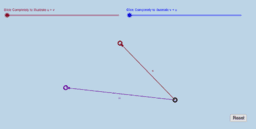Activity

Tim Brzezinski

•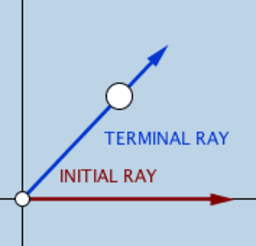### Angles in Standard Position

Activity

Tim Brzezinski

•### Vector Projections (1)

Activity

Tim Brzezinski

•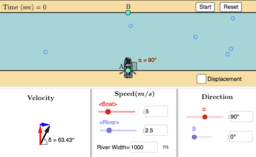### Relative velocity: Boat problem

Activity

Juan Carlos Ponce Campuzano

•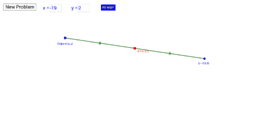### Endpoint (x,y) of a Segment Given Midpoint & Other Endpoint

Activity

Tim Brzezinski

•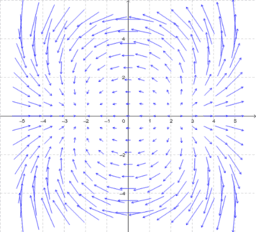### Vector Fields

Activity

Juan Carlos Ponce Campuzano

•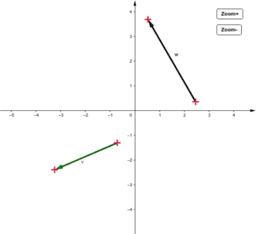Activity

Juan Carlos Ponce Campuzano

•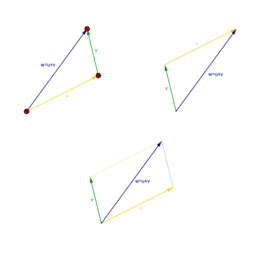Activity

•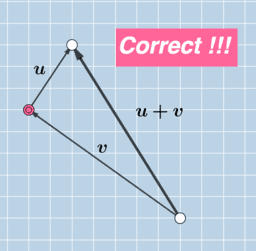Activity

Tim Brzezinski

•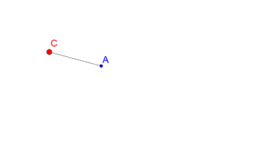### Demo: constrain a Segment to 15° intervals

Activity

Michael Borcherds

•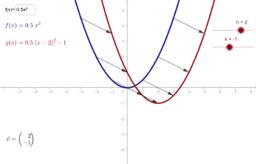### Translation using vector notation

Activity

•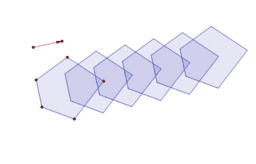### Translations along vector

Activity

•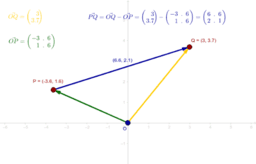### Vector subtraction and bound vectors

Activity

•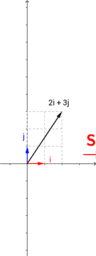### Matrix and Linear Transformation

Activity

Anthony OR 柯志明

•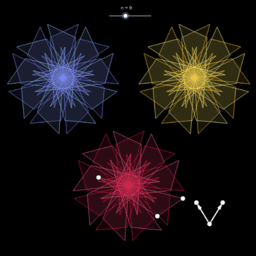Activity

•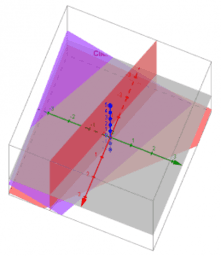### Linear Algebra

Book

Kevin Hopkins

•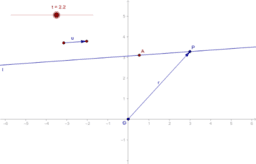### Equation of a line through point along vector

Activity

•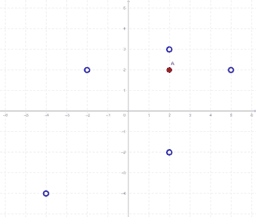### Points and vectors

Activity

•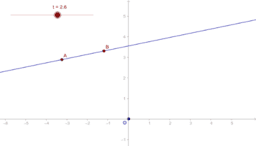### Equation of a line through two points

Activity

•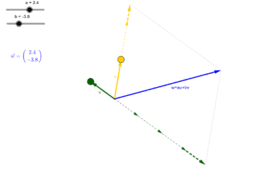### w defined by the basis vectors u and v.

Activity

•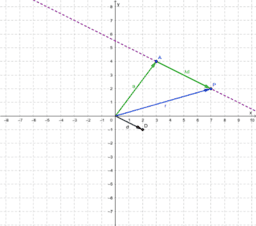### Vector equation of a line (2D)

Activity

•### Linear transformations

Activity

•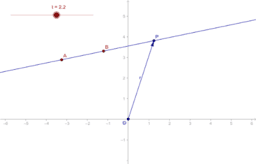### Equation of a line, through two points

Activity

•